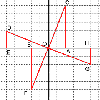#### You may also like### 8 Methods for Three by One

This problem in geometry has been solved in no less than EIGHT ways by a pair of students. How would you solve it? How many of their solutions can you follow? How are they the same or different? Which do you like best?### Reflect Again

Follow hints to investigate the matrix which gives a reflection of the plane in the line y=tanx. Show that the combination of two reflections in intersecting lines is a rotation.### The Matrix

Investigate the transfomations of the plane given by the 2 by 2 matrices with entries taking all combinations of values 0. -1 and +1.

# Rots and Refs

##### Age 16 to 18Challenge Level

See the problem 'The Matrix' for an explanation of how a transformation of the plane is given by a matrix and how you can find the image of a point by multiplying its vector by the matrix of the transformation.